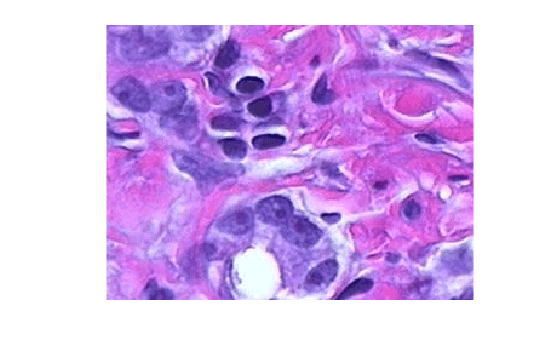# deltaE

Color difference based on CIE76 standard

## Syntax

``dE = deltaE(I1,I2)``
``dE = deltaE(I1,I2,'isInputLab',isLab)``

## Description

example

````dE = deltaE(I1,I2)` calculates the color difference between two RGB images or sets of colors using the CIE76 standard.```

example

````dE = deltaE(I1,I2,'isInputLab',isLab)` also specifies whether the input color data is in the RGB color space or the L*a*b* color space.```

## Examples

collapse all

Specify two RGB color values.

```pureRed = uint8([255 0 0]); darkRed = uint8([255 10 50]);```

Calculate the color difference of the colors.

`dE = deltaE(pureRed,darkRed)`
```dE = single 18.6206 ```

Read a color image into the workspace.

```I1 = imread('peppers.png'); imshow(I1)```Alter the local color contrast in the image.

```I2 = localcontrast(I1); imshow(I2)```Calculate the color difference of the images.

`dE = deltaE(I1,I2);`

Display the color difference as an image. The maximum value of `dE` exceeds the range [0, 1] expected of images of data type `single`, so display the image using the full display range of the data. Bright pixels indicate a large color difference and therefore a larger amount of contrast enhancement.

`imshow(dE,[])`Read and display an image of tissue stained with hemotoxylin and eosin (H&E).

```he = imread('hestain.png'); imshow(he)```Convert the image to the L*a*b* color space.

`lab = rgb2lab(he);`

Make a copy of the image, then increase the signal of the a* channel. Red tones in the image become more saturated while the image overall brightness and the blue tones are unchanged.

```lab2 = lab; scaleFactor = 1.2; lab2(:,:,2) = scaleFactor*lab(:,:,2);```

Calculate the color difference of the original and enhanced image in the L*a*b* color space.

`dE = imcolordiff(lab,lab2,'isInputLab',true);`

Display the color difference as an image. Scale the display range to match the range of pixel values in `dE`. Bright regions indicate the greatest color difference and correspond with the pink regions of tissue.

`imshow(dE,[])`## Input Arguments

collapse all

First set of color data, specified as an m-by-n-by-3 numeric array representing an image or a c-by-3 numeric matrix representing a set of c colors. `I1` and `I2` must be the same size with values in the same color space.

By default, the `deltaE` function interprets the color data as RGB color values. To calculate the color difference in the L*a*b* color space, specify the `isLab` argument as `true`. L*a*b* color values can be of data type `single` or `double` only.

Data Types: `single` | `double` | `uint8` | `uint16`

Second set of color data, specified as an m-by-n-by-3 numeric array representing an image or a c-by-3 numeric matrix representing a set of c colors. `I1` and `I2` must be the same size with values in the same color space.

By default, the `deltaE` function interprets the color data as RGB color values. To calculate the color difference in the L*a*b* color space, specify the `isLab` argument as `true`. L*a*b* color values can be of data type `single` or `double` only.

Data Types: `single` | `double` | `uint8` | `uint16`

Color values are in the L*a*b* color space, specified as a numeric or logical `0` (`false`) or `1` (`true`).

## Output Arguments

collapse all

Color difference (delta E), returned as one of the following.

• An m-by-n matrix when the input color data `I1` and `I2` represent images

• A c-element column vector when `I1` and `I2` represent a set of c colors

If `I1` or `I2` is of data type `double`, then `dE` is of data type `double`. Otherwise, `dE` is of data type `single`.

Data Types: `single` | `double`

## Tips

• To improve the accuracy of the color difference calculation, use the `imcolordiff` function. This function follows the CIE94 and CIEDE2000 standards and offers parameters to improve perceptual uniformity for different applications.

## Version History

Introduced in R2020b